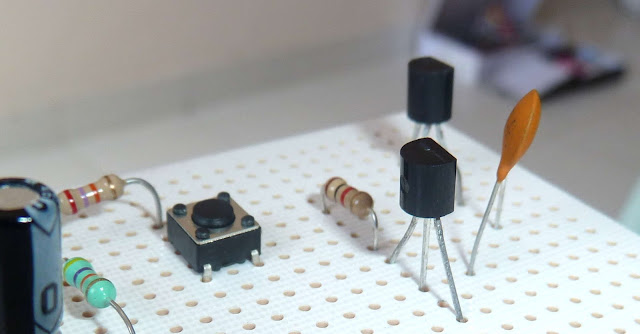### Design of Electronic Devices & Circuits - MCQ from AMIE exams (Summer 2018)Choose the correct answer for the following (10 x 2)

1. When a differential amplifier is operated single ended,
(a) The output is grounded
(b) One input is grounded, and a signal is applied to the other
(c) Both inputs are connected together
(d) The output is not inverted

2. If ADM = 3500; ACM = 0.35, the CMRR is_______
(a) 1225
(b) 10,000
(c) 80 dB

3. Negative feedback _____
(a) Increases the input and output impedances
(b) Increases the input impedance and bandwidth
(c) Decreases the output impedance and bandwidth
(d) Does not affect impedance or bandwidth.

4. The cut-off frequency of a first-order low-pass filter for RI = 2.5 kW and CI = 0.05 µF is
(a) 1.273 kHz
(b) 12.73 kHz
(c) 127.3 kHz
(d) 127.3 Hz

5. Which among the following does not belong to the category of LC oscillators?
(a) Hartley oscillator
(b) Colpitt’s oscillator
(c) Clapp oscillator
(d) Wein bridge oscillator

6. Which among the following is not an advantage of RC coupled amplifiers?
(a) High fidelity
(d) No core distortion
(c) No impedance matching
(d) Wide frequency response

7. Which among the below stated parameters gets affected due to drift region in the power transistor?
(a) Breakdown voltage
(b) On-state losses
(c) Switching time
(d) All of the above

8. After passing through which circuit network, does the signal Xd (output signal obtained by taking the difference of two input signals) get multiplied by‘-l’?
(a) Amplifier circuit
(b) Feedback network
(c) Mixing network
(d) Sampling network

9. Circuit that make use of techniques for varying the frequency of the carrier oscillator in accordance with the modulating signal arc referred to as
(a) Direct FM
(b) Indirect FM
(c) Phase modulation
(d) Demodulation

10. Which of the following is not a basic element of a phase-locked loop circuit?
(a) Phase detector
(d) Parallel tuned circuit
(c) Voltage-controlled oscillator
(d) Low-pass filter.

1. (b) All the voltages are measured with respect to the ground voltage. The ground voltage is considered to be 0 V. As the name suggested, a single-ended differential amplifier amplifies the signal that is given through only one of the input.

2. (d)
= 3500/0.35
= 10,000
= 20log(10,000)
= 80 dB

3. (b)

4. (a)
f = 1/2πR1C1
= 1/(2)(3.14)(2.5x103)(0.05 x 10-6)
= 1273 Hz
= 1.273 kHz

5.  (d)

6. (c)

• It makes use of resistors and capacitors which are not expensive, so the cost of RC coupled amplifier is low.
• The circuit is very compact and extremely light.
• The frequency response of RC coupled amplifier is excellent.
• It offers a constant gain over a wide frequency range.
• It has low voltage and power gain.
• It is unsuitable for low frequency application.
• It has the tendency to become noisy with times.
• It has narrow bandwidth.
7. (d)

8. (c)

9. (a) In direct FM, as the information signal is received, the instantaneous frequency is directly varied with the information signal. While an oscillator is used to change the carrier frequency where its resonant frequency is determined by variable components.

10. (d) A phase-locked loop is a control system that generates an output signal whose phase is related to the phase of an input signal. The circuit can track an input frequency, or it can generate a frequency that is a multiple of the input frequency. The basic elements of a PLL circuit are phase comparator/detector, a loop filter, a voltage controlled oscillator (VCO).

---

The study material for AMIE/Junior Engineer exams is available at https://amiestudycircle.com